# 图像频域滤波与傅里叶变换

2019/04/10 10:10

## 1、频率滤波

subplot(2,1,1);
imshow(img), title('原始图像');
line1 = img(1, :);
line2 = img(2, :);
line3 = img(3, :);
subplot(2,1,2);
hold on
plot(line1, 'r');
plot(line2, 'g');
plot(line3, 'b');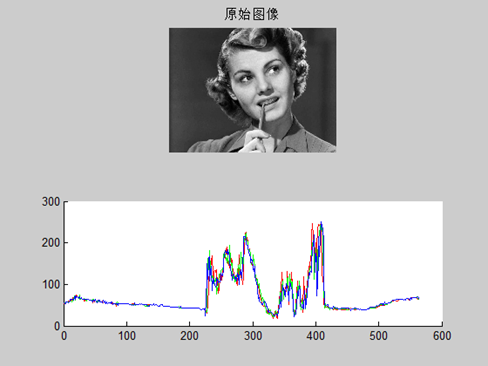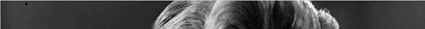## 2、图像傅里叶变换

imgPath = 'E:\opencv_pic\src_pic\pic4.bmp';
img=rgb2gray(img);

f=fft2(im2double(img)); %FFT
F=f; %FFT频谱
T=log(F+1); %频谱对数变换
subplot(1,2,1),imshow(img),title('原始图像');
subplot(1,2,2),imshow(T,[]),title('原始图像其频谱图');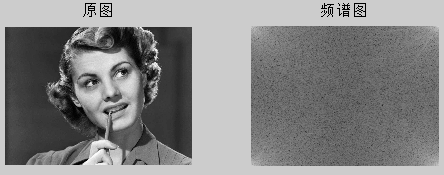Y = fftshift(X) 通过将零频分量移动到数组中心，重新排列傅里叶变换 X。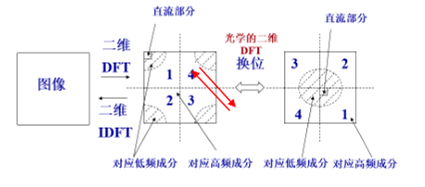a =
1     2     3     4
6     7     8     9
>> fftshift(a)
ans =
8     9     6     7
3     4     1     2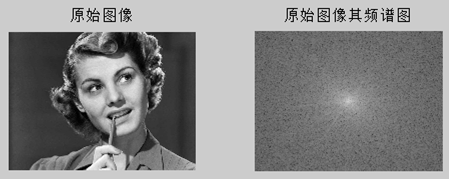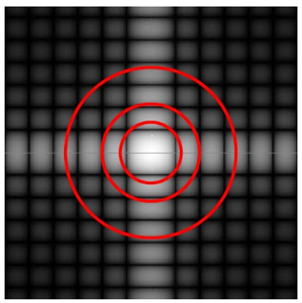## 3、频域滤波

G=imnoise(img,'gaussian', 0, 0.05);%模拟均值为0方差为0.05的高斯噪声，
H=fft2(im2double(G)); %FFT
H=fftshift(H); %FFT频谱平移
T=log(abs(H)); %频谱对数变换
subplot(2,2,1), imshow(G),title('添加高斯噪声图像');
subplot(2,2,2),imshow(T, []),title('频谱图');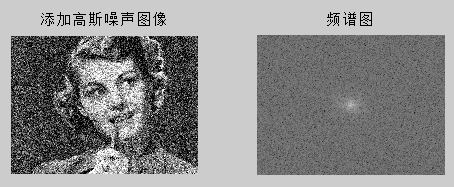img2 = (ifft2(ifftshift(H))); %===频域的图反变换到空域

img3 = im2uint8(mat2gray(img2)); %===取其灰度图

subplot(2,2,3),imshow(img3);

title('anti-Fourier');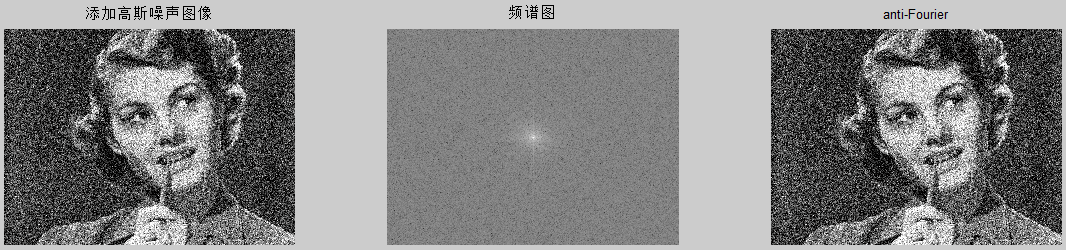1)低通滤波器：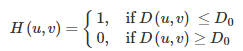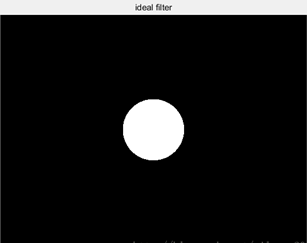2)高通滤波器: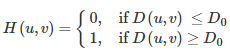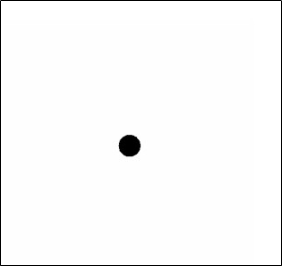3）高斯低通滤波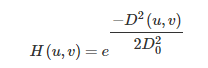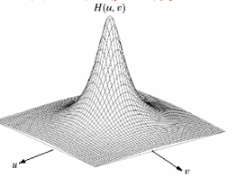4）高斯高通滤波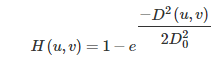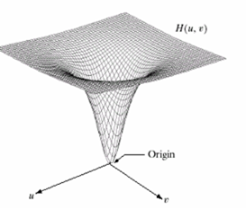S=fftshift(fft2(G)); %G是添加高斯噪声的图像
[M,N]=size(S);
d0=5; %GLPF滤波，d0=5，15，30
n1=floor(M/2);
n2=floor(N/2);
for i=1:M
for j=1:N
d=sqrt((i-n1)^2+(j-n2)^2);
h=1*exp(-1/2*(d^2/d0^2));
S(i,j)=h*S(i,j);
end
end

S=ifftshift(S);
S=uint8(ifft2(S));
subplot(2,2,4),imshow(S),title('高斯低通滤波图像');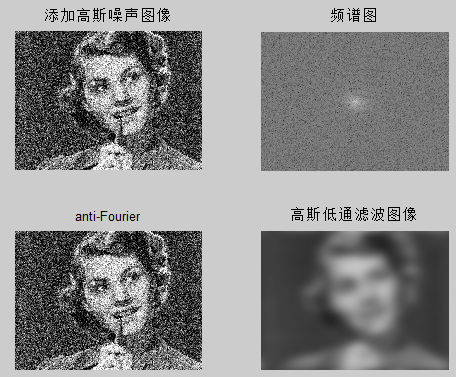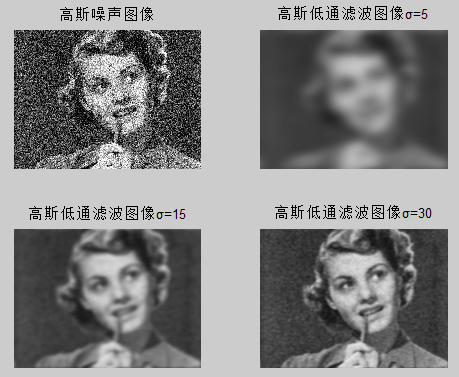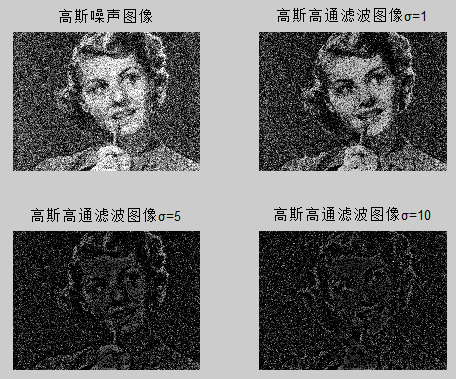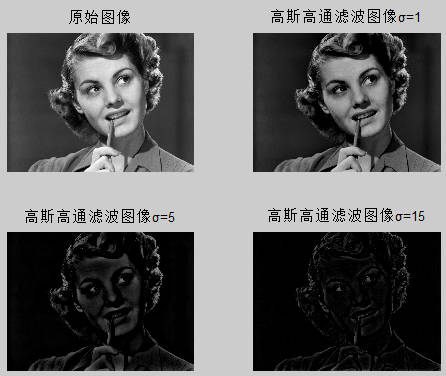## 5、参考文献：

1、《数字图像处理与机器视觉》

2、傅里叶变换在图像处理中的应用

https://www.cnblogs.com/Lynn0101/p/9892469.html

3、fftshift

https://ww2.mathworks.cn/help/matlab/ref/fftshift.html

https://www.cnblogs.com/pingwen/p/12442257.html

0
0 收藏

### 作者的其它热门文章0 评论
0 收藏
0